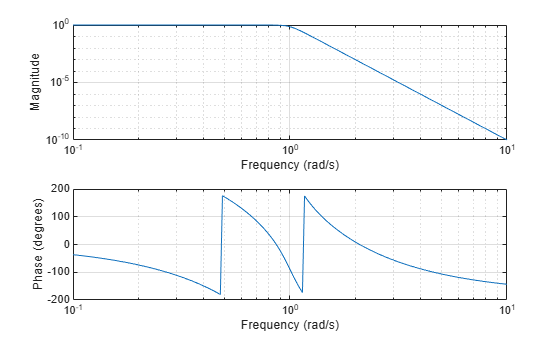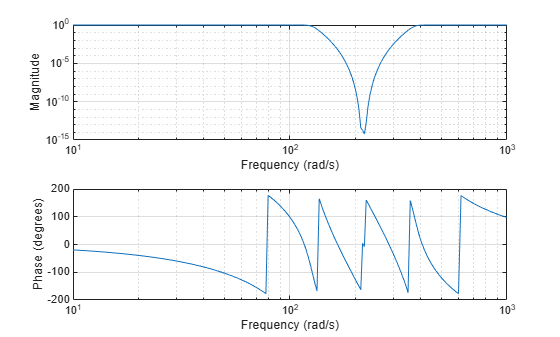# lp2bs

Transform lowpass analog filters to bandstop

## Syntax

``[bt,at] = lp2bs(b,a,Wo,Bw)``
``[At,Bt,Ct,Dt] = lp2bs(A,B,C,D,Wo,Bw)``

## Description

example

````[bt,at] = lp2bs(b,a,Wo,Bw)` transforms an analog lowpass filter prototype given by polynomial coefficients (specified by row vectors `b` and `a`) into a bandstop filter with center frequency `Wo` and bandwidth `Bw`. The input system must be an analog filter prototype.```
````[At,Bt,Ct,Dt] = lp2bs(A,B,C,D,Wo,Bw)` converts the continuous-time state-space lowpass filter prototype (specified by matrices `A`, `B`, `C`, and `D`) to a bandstop filter with center frequency `Wo` and bandwidth `Bw`. The input system must be an analog filter prototype.```

## Examples

collapse all

Design a 10th-order lowpass Butterworth analog filter prototype.

```n = 10; [z,p,k] = buttap(n);```

Convert the prototype to transfer function form. Display its magnitude and frequency responses.

```[b,a] = zp2tf(z,p,k); freqs(b,a)```Transform the prototype to a bandstop filter with a stopband from 20 Hz to 60 Hz. Specify the center frequency and bandwidth in rad/s.

```fl = 20; fh = 60; Wo = 2*pi*sqrt(fl*fh); % center frequency Bw = 2*pi*(fh-fl); % bandwidth [bt,at] = lp2bs(b,a,Wo,Bw);```

Display the magnitude and frequency responses of the transformed filter.

`freqs(bt,at)`## Input Arguments

collapse all

Prototype numerator and denominator coefficients, specified as row vectors. `b` and `a` specify the coefficients of the numerator and denominator of the prototype in descending powers of s:

`$\frac{B\left(s\right)}{A\left(s\right)}=\frac{b\left(1\right){s}^{n}+\cdots +b\left(n\right)s+b\left(n+1\right)}{a\left(1\right){s}^{m}+\cdots +a\left(m\right)s+a\left(m+1\right)}$`

Data Types: `single` | `double`

Prototype state-space representation, specified as matrices. The state-space matrices relate the state vector x, the input u, and the output y through

`$\begin{array}{l}\stackrel{˙}{x}=Ax+Bu\\ y=Cx+Du\end{array}$`

Data Types: `single` | `double`

Center frequency, specified as a scalar. For a filter with lower band edge `w1` and upper band edge `w2`, use `Wo` = sqrt(`w1`*`w2`). Express `Wo` in units of rad/s.

Data Types: `single` | `double`

Bandwidth, specified as a scalar. For a filter with lower band edge `w1` and upper band edge `w2`, use `Bw` = `w2``w1`. Express `Bw` in units of rad/s.

Data Types: `single` | `double`

## Output Arguments

collapse all

Transformed numerator and denominator coefficients, returned as row vectors.

Transformed state-space representation, returned as matrices.

## Algorithms

`lp2bs` transforms analog lowpass filter prototypes with a cutoff angular frequency of 1 rad/s into bandstop filters with the desired bandwidth and center frequency. The transformation is one step in the digital filter design process for the `butter`, `cheby1`, `cheby2`, and `ellip` functions.

`lp2bs` is a highly accurate state-space formulation of the classic analog filter frequency transformation. If a bandstop filter has center frequency ω0 and bandwidth Bw, the standard s-domain transformation is

`$s=\frac{p}{Q\left({p}^{2}+1\right)}$`

where Q = ω0/Bw and p = s0. The state-space version of this transformation is

`$Q=\frac{{\omega }_{0}}{{B}_{w}}$`
`$At=\left[\frac{{\omega }_{0}}{Q\cdot {A}^{-1}}\text{\hspace{0.17em}}\text{\hspace{0.17em}}{\omega }_{0}\cdot \text{eye}\left(ma\right);-{\omega }_{0}\cdot \text{eye}\left(ma\right)\text{\hspace{0.17em}}\text{\hspace{0.17em}}\text{zeros}\left(ma\right)\right]$`
`$Bt=-\left[\frac{{\omega }_{0}}{Q\left(A\B\right)};\text{zeros}\left(ma,n\right)\right]$`
`$Ct=\left[\frac{C}{A}\text{\hspace{0.17em}}\text{\hspace{0.17em}}\text{\hspace{0.17em}}\text{zeros}\left(mc,ma\right)\right]$`
`$Dt=D-C/A\cdot B$`

`lp2bs` can perform the transformation on two different linear system representations: transfer function form and state-space form. See `lp2bp` for a derivation of the bandpass version of this transformation.